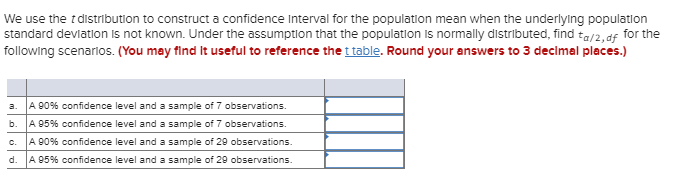Home / Answered Questions / Other / we-use-the-t-distribution-to-construct-a-confidence-interval-for-the-population-mean-when-the-underl-aw915

# (Solved): We Use The T Distribution To Construct A Confidence Interval For The Population Mean When The Underl...We use the t distribution to construct a confidence Interval for the population mean when the underlying population standard deviation is not known. Under the assumption that the population is normally distributed, find ta/2,df for the following scenarios. (You may find it useful to reference the t table. Round your answers to 3 decimal places.) a. A 90% confidence level and a sample of 7 observations. b. A 95% confidence level and a sample of 7 observations. c. A 90% confidence level and a sample of 29 observations. d. A 95% confidence level and a sample of 29 observations.

We have an Answer from Expert